Saturday, August 15, 2020
Home > CBSE Class 12 > NCERT Solutions for Class 12 Maths Determinants Miscellaneous Exercise

# NCERT Solutions for Class 12 Maths Determinants Miscellaneous Exercise# NCERT Solutions for Class 12 Maths Determinants Miscellaneous Exercise

Hi Students, Welcome to Amans Maths Blogs (AMB). In this post, you will get the NCERT Solutions for Class 12 Maths Determinants Miscellaneous Exercise. This NCERT Solutions can be downloaded in PDF file. The downloading link is given at last.

NCERT Solutions for Class 12 Maths are not only the solutions of Maths exercise but it builds your foundation of other important subjects. Getting knowledge of depth concept of CBSE Class 12th Maths like Algebra, Calculus, Trigonometry, Coordinate Geometry help you to understand the concept of Physics and Physical Chemistry.

CBSE Class 12th is an important school class in your life as you take some serious decision about your career. And out of all subjects, Maths is an important and core subjects. So CBSE NCERT Solutions for Class 12th Maths is major role in your exam preparation as it has detailed chapter wise solutions for all exercise.

As we know that all the schools affiliated from CBSE follow the NCERT books for all subjects. You can check the CBSE NCERT Syllabus. Thus, NCERT Solutions helps the students to solve the exercise questions as given in NCERT Books.

## NCERT Solutions for Class 12 Maths Determinants Miscellaneous Exercise

NCERT Solutions for Class 12 Maths Determinants Miscellaneous Exercise: Ques No 1.Prove that the determinant,is independent of θ.

NCERT Solutions:Expanding along R1, we get

Δ = x(-x2 – 1) – sinθ(-xsinθ – cosθ) + cosθ(-sinθ + xcosθ)

= -x3 – x + xsin2θ + sinθcosθ – sinθcosθ + xcos2θ

= -x3 – x + x(sin2θ + cos2θ) = -x3 – x + x

= -x3

= Independent of θ.

NCERT Solutions for Class 12 Maths Determinants Miscellaneous Exercise: Ques No 2.Without expanding the determinant, prove that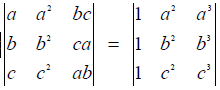NCERT Solutions:NCERT Solutions for Class 12 Maths Determinants Miscellaneous Exercise: Ques No 3.Evaluate.

NCERT Solutions:NCERT Solutions for Class 12 Maths Determinants Miscellaneous Exercise: Ques No 4.If a, b and c are real numbers,and Show that either a + b + c = 0 or a = b = c.

NCERT Solutions:NCERT Solutions for Class 12 Maths Determinants Miscellaneous Exercise: Ques No 5.Solve the equation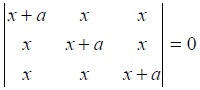, a ≠ 0.

NCERT Solutions:NCERT Solutions for Class 12 Maths Determinants Miscellaneous Exercise: Ques No 6.Prove that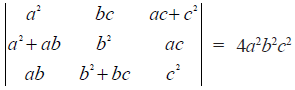.

NCERT Solutions:NCERT Solutions for Class 12 Maths Determinants Miscellaneous Exercise: Ques No 7.If A–1 =and B =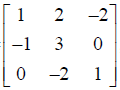, find (AB)-1.

NCERT Solutions: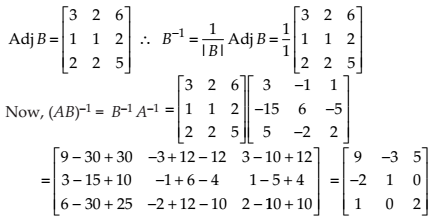NCERT Solutions for Class 12 Maths Determinants Miscellaneous Exercise: Ques No 8.Let A =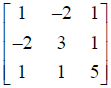, Verify that (i) [adj A]–1 = adj (A–1) (ii) (A–1)–1 = A

NCERT Solutions: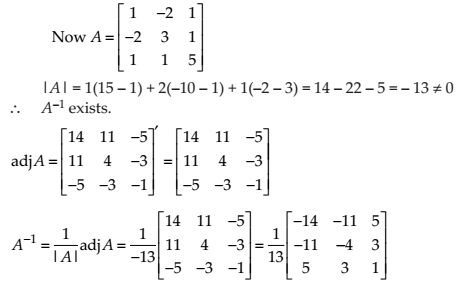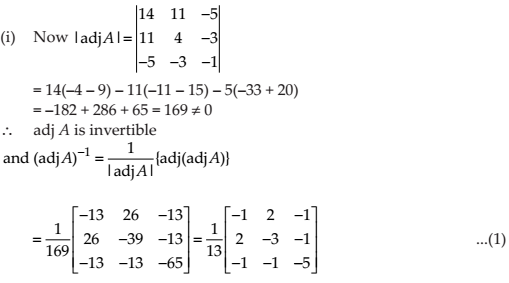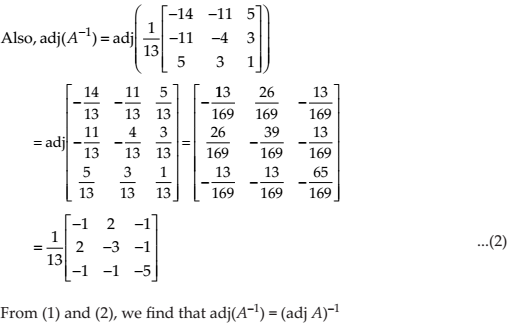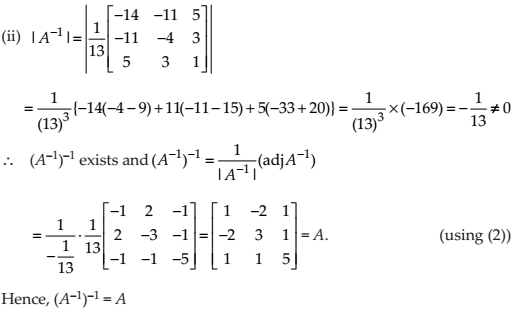NCERT Solutions for Class 12 Maths Determinants Miscellaneous Exercise: Ques No 9.Evaluate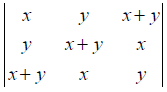.

NCERT Solutions: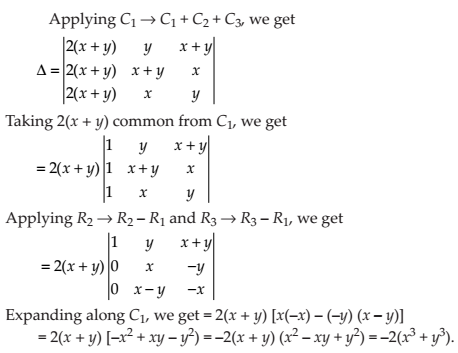NCERT Solutions for Class 12 Maths Determinants Miscellaneous Exercise: Ques No 10.Evaluate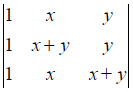NCERT Solutions:Using properties of determinants in Exercises 11 to 15, prove that:

NCERT Solutions for Class 12 Maths Determinants Miscellaneous Exercise: Ques No 11.NCERT Solutions: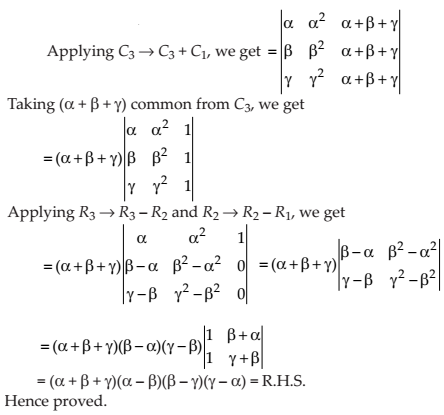NCERT Solutions for Class 12 Maths Determinants Miscellaneous Exercise: Ques No 12.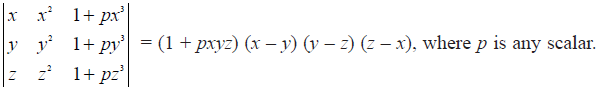NCERT Solutions: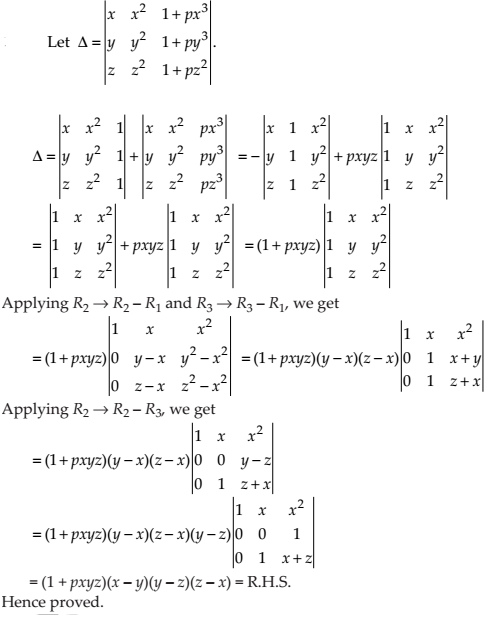NCERT Solutions for Class 12 Maths Determinants Miscellaneous Exercise: Ques No 13.NCERT Solutions:NCERT Solutions for Class 12 Maths Determinants Miscellaneous Exercise: Ques No 14.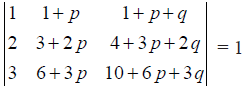NCERT Solutions:NCERT Solutions for Class 12 Maths Determinants Miscellaneous Exercise: Ques No 15.NCERT Solutions:NCERT Solutions for Class 12 Maths Determinants Miscellaneous Exercise: Ques No 16.Solve the system of equations

2/x + 3/y + 10/z = 4,

4/x – 6/y + 5/z = 1,

6/x + 9/y – 20/z = 2

NCERT Solutions: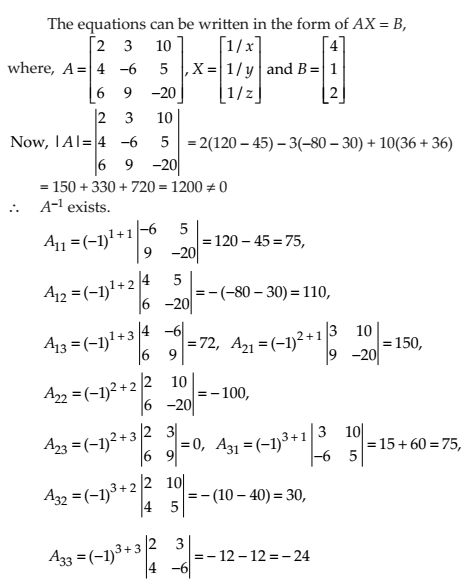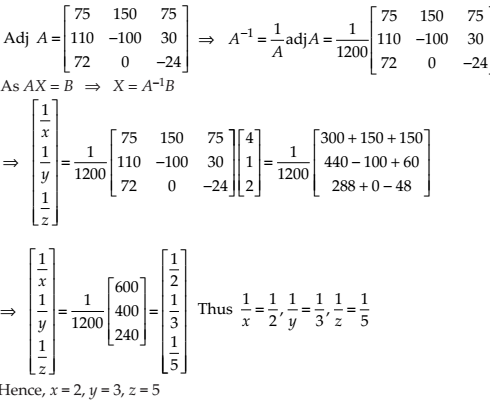NCERT Solutions for Class 12 Maths Determinants Miscellaneous Exercise: Ques No 17.If a, b, c, are in A.P, then the determinantis

(A) 0

(B) 1

(C) x

(D) 2x

NCERT Solutions:NCERT Solutions for Class 12 Maths Determinants Miscellaneous Exercise: Ques No 18.If x, y, z are nonzero real numbers, then the inverse of matrix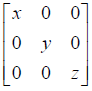is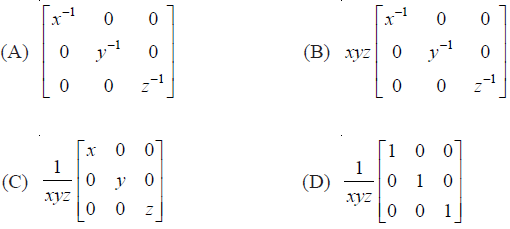NCERT Solutions:NCERT Solutions for Class 12 Maths Determinants Miscellaneous Exercise: Ques No 19.Let A =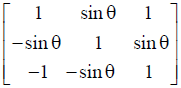, where 0 ≤ θ ≤ 2π. Then

(A) Det (A) = 0

(B) Det (A) ∈ (2, ∞)

(C) Det (A) ∈ (2, 4)

(D) Det (A) ∈ [2, 4]

NCERT Solutions:error: Content is protected !!